# 我的机器学习课笔记 #1-绪论与模型评估

[TOC]

## 没有免费午餐定理（no free lunch theorem, or NFL）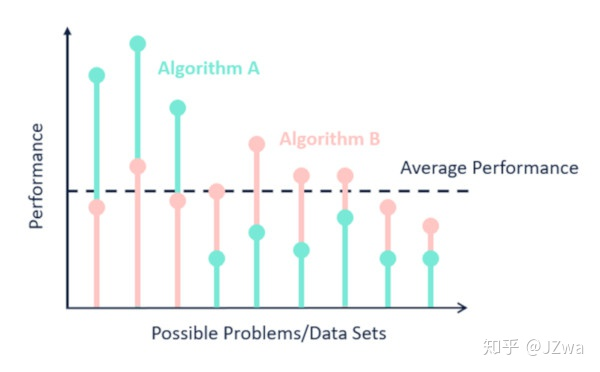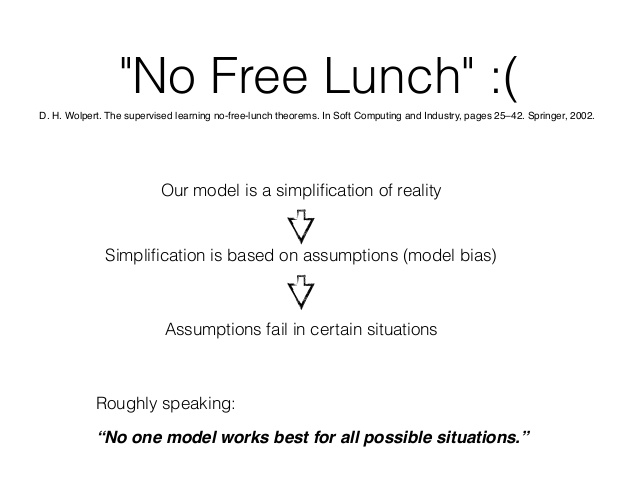## 模型评估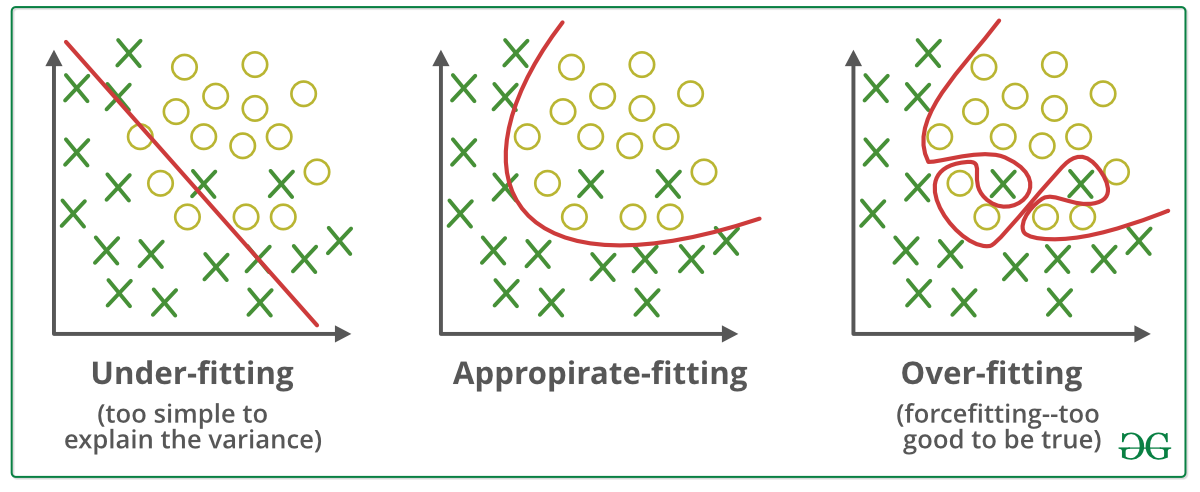### 如何得到训练集和测试集？

• 留出法

最常用，就是把数据集的划分成两个互斥的集合，一个训练一个预测。

• 交叉验证法

小样本适用，因为会带来更大的计算开销。K折交叉验证指的是将数据集随机分成K份，进行K次训练和预测，每次训练选取K-1份作为训练集，每次预测选取剩下那1份作为测试集。

• 自助法

适用于集成学习，不会减少训练集的数据数量，但会改变数据集的分布。就是将一个拥有m个数据的数据集进行放回抽样，抽m次出来得到的就是训练集，用所有数据去掉训练集就是测试集，易知训练集的数量是m，但里面会有重复的数据。

### 使用哪种误差函数？

#### 混淆矩阵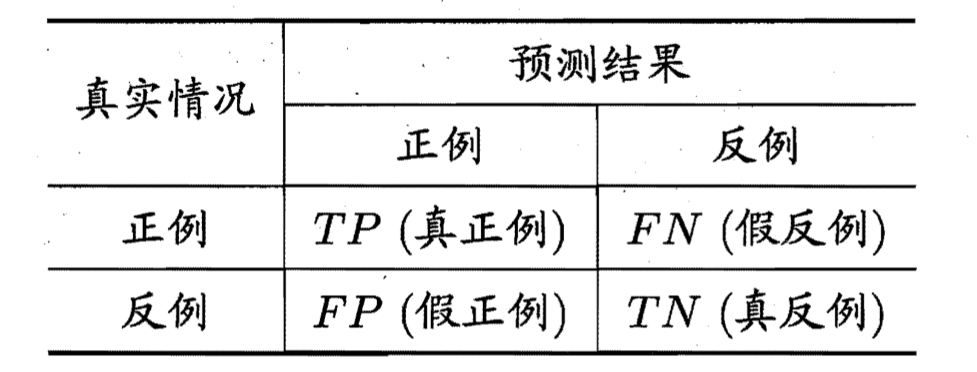#### 查准率、查全率、P-R曲线、F1

$$查准率、精确率：Precise=\frac{TP}{TP+FP}$$

$$查全率、召回率：Recall=\frac{TP}{TP+FN}$$

P-R曲线就是纵轴P，横轴R的曲线。分类问题一般会对测试数据得出一个预测概率，从高到低排序，可以手动选择最高的k个作为预测正例，在k不确定的时候，查准率和查全率就会呈现P-R曲线。假如k很大，那么查全率就会高，查准率就会低，因为把很多低概率的结果都判成正例。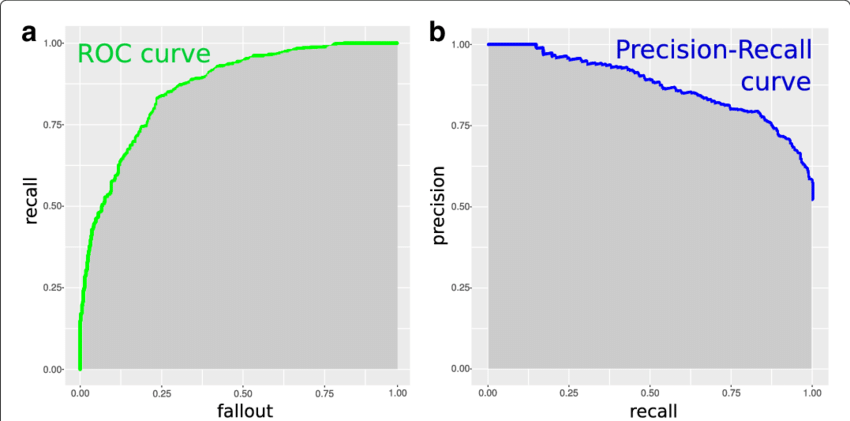$$\frac{1}{F1} = \frac{1}{2} (\frac{1}{P}+\frac{1}{R})$$

$$\frac{1}{F_\beta} = \frac{1}{1+\beta^2} (\frac{1}{P}+\frac{\beta^2}{R})$$

• 去程速度为30 mph
• 返程时交通有一些拥堵，所以速度为10 mph
• 去程和返程走的是同一路线，也就是说距离一样（5英里）

#### ROC和AUC

$$FPR=\frac{FP}{TN+FP}$$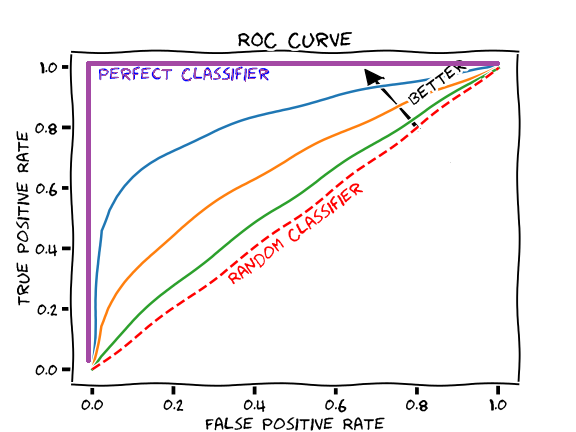## 模型性能分析

$$泛化误差=偏差(bias)+方差(var)+噪声(noise)$$

• 偏差指的是模型算法本身的拟合能力，是预测结果与真实结果的偏离程度
• 方差指的是模型对于相同大小的训练集的学习结果的抖动。
• 噪声是和数据集本身有关的泛化误差的下界，即学习本身的难度。

Machine Learning’s No Free Lunch Theorem Explained (analyticsindiamag.com)

No free lunch theorem-没有免费的午餐 - 知乎 (zhihu.com)